Refer to our Texas Go Math Grade 2 Answer Key Pdf to score good marks in the exams. Test yourself by practicing the problems from Texas Go Math Grade 2 Module 8 Assessment Answer Key.

Concepts and Skills

Break apart the number you are subtracting. Write the difference. (TEKS 2.4.B)

Question 1.
71 – 6 = ___________
71 – 6 = 65
Explanation:
The partners of 6 are 1 and 5
71 – 1 = 70
70 – 5 = 65

Question 2.
83 – 7 = ____________
83 – 7 = 76
Explanation:
The partners of 7 are 3 and 4
83 – 3 = 80
80 – 4 = 76

Question 3.
87 – 18 = ___________
87 – 18 = 69
Explanation:
The partners of 18 are 7 and 11
87 – 7 = 80
80 – 11= 69

Question 4.
79 – 12 = ___________
79 – 12 = 67
Explanation:
The partners of 12 are 9 and 3
79 – 9 = 70
70 – 3 = 67

Draw a quick picture to solve. Write the difference. (TEKS 2.4.B)

Question 5.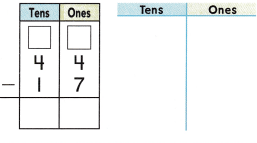Explanation:
The line represents the ten
and the dot represents the ones

Question 6.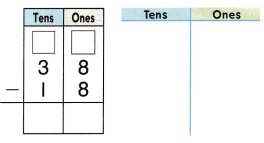Explanation:
The line represents the ten
and the dot represents the ones

Question 7.
Label the model. Write a number sentence with a ☐ for the unknown number. Solve. (TEKS 2.4.C)
Charlie sees 35 geese in a field. He sees 12 geese in the sky. How many more geese does he see ¡n the field than in the sky?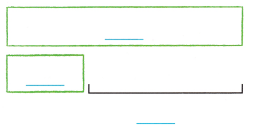__________ more geese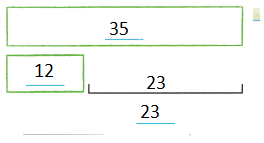Explanation:
Charlie sees 35 geese in a field.
He sees 12 geese in the sky.
35 – 12 = 23
23 more geese that  he see in the field than in the sky

Fill in the bubble for the correct answer choice.

Question 8.
Cindy counts 53 shells on the beach. 18 shells wash away. How many shells are on the beach now? (TEKS 2.4.B)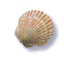(A) 45
(B) 61
(C) 35
Explanation:
Cindy counts 53 shells on the beach.
18 shells wash away.
53 – 18 = 35
35 shells are on the beach now

Question 9.
There are 58 books at the store. 32 books are on a shelf and the rest are on a table. How many books are on the table? (TEKS 2.4.B)
(A) 16
(B) 26
(C) 56
Explanation:
There are 58 books at the store.
32 books are on a shelf and the rest are on a table.
58 – 32 = 26
26 books are on the table

Question 10.
Children fly 61 kites at the festival. 7 kites fall to the ground. How many kites are still flying? (TEKS 2.4.B)(A) 54
(B) 66
(C) 13
Explanation:
Children fly 61 kites at the festival.
7 kites fall to the ground.
61 – 7 = 54
54 kites are still flying

Question 11.
There are 72 crayons in the box. Matt and Devon each take 18 crayons out of the box. How many crayons are in the box now? (TEKS 2.4.B)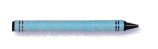(A) 90
(B) 36
(C) 54